Here we are providing Online Education for Constructions Class 9 Extra Questions Maths Chapter 11 with Answers Solutions, Extra Questions for Class 9 Maths was designed by subject expert teachers. https://ncertmcq.com/extra-questions-for-class-9-maths/

## Extra Questions for Class 9 Maths Constructions with Answers Solutions

Extra Questions for Class 9 Maths Chapter 11 Constructions with Solutions Answers

### Constructions Class 9 Extra Questions Very Short Answer Type

Construction Class 9 Extra Questions Question 1.
Draw a line segment AB = 8 cm. Draw $$\frac{1}{3}$$ part of it. Measure the length of $$\frac{1}{3}$$ part of AB.
Solution: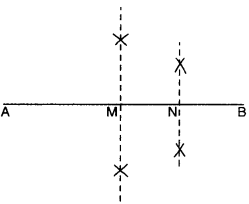Steps of Construction :

1. Draw a line segment AB = 8 cm.
2. Draw its perpendicular bisector and let it intersect AB in M.
3. Draw the perpendicular bisector of MB and let it intersect AB in N. Thus, AN = $$\frac{1}{3}$$ of AB = 6 cm.

Class 9 Construction Extra Questions Question 2.
Why we cannot construct a ∆ABC, if ∠A = 60°, AB = 6 cm and AC + BC = 5 cm but construction of ∆ABC is possible if ∠A = 60°, AB = 6 cm and AC – BC = 5 cm ?
Solution:
We know that, by triangle inequality property, construction of triangle is possible if sum of two sides of a triangle is greater than the third side. Here, AC + BC = 5 cm which is less than AB (6 cm) Thus, ∆ABC is not possible.
Also, by triangle inequality property, construction of triangle is possible, if difference of two sides of a triangle is less than the third side
Here, AC – BC = 5 cm, which is less than AB (6 cm)
Thus, ∆ABC is possible.

Constructions Class 9 Extra Questions Question 3.
Construct an angle of 90° at the initial point of the given ray.
Solution: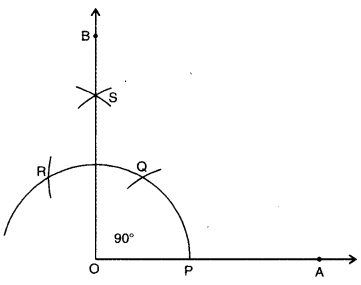Steps of Construction :

1. Draw a ray OA.
2. With O as centre and any convenient radius, draw an arc, cutting OA at P.
3. With P as centre and same radius, draw an arc cutting the arc drawn in step 2 at Q.
4. With Q as centre and the same radius as in steps 2 and 3, draw an arc, cutting the arc drawn in step 2 at R.
5. With Q and R as centres and same radius, draw two arcs, cutting each other in S.
6. Join OS and produce to B. Thus, ∠AOB is the required angle of 90°

Construction Extra Questions Class 9 Question 4.
Draw a straight angle. Using compass bisect it. Name the angles obtained.
Solution:Steps of Construction :

1. Draw any straight angle (say ∠AOC).
2. Bisect ∠AOC and join BO.
3. ∠AOB is the required bisector of straight angle AOC.

Class 9 Maths Constructions Extra Questions Question 5.
Draw any reflex angle. Bisect it using compass. Name the angles so obtained.
Solution: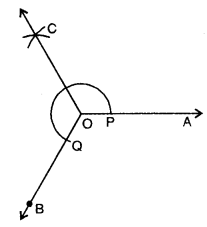Steps of Construction :

1. Let ∠AOB be any reflex angle.
2. With O as centre and any convenient radius, draw an arc cutting OA in P and OB in Q.
3. With P and Q as centres, draw two arcs of radius little more than half of it and let they intersect each other in C. Join OC. Thus, OC is the required bisector. Angles so obtained are ∠AOC and ∠COB.

### Constructions Class 9 Extra Questions Short Answer Type 1 and 2

Class 9 Maths Chapter 11 Extra Questions Question 1.
Construct a triangle whose sides are in the ratio 2 : 3 : 4 and whose perimeter is 18 cm.
Solution:Steps of Construction :

1. Draw a line segment AB =18 cm.
2. At A, construct an acute angle ∠BAX (< 90°).
3. Mark 9 points on AX, such that AA1 = A1A2 = A2A3 = A3A4 = A4A5 = A5A6
= A6A7 = A7A8 = A8A9.
4. Join A9B.
5. From A2 and A5, draw A2M || A5N || A9B, intersecting AB in M and N respectively.
6. With M as centre and radius AM, draw an arc.
7. With N as centre and radius NB, draw another arc intersecting the previous arc at L.
8. Join LM and LN. Thus, ∆LMN is the required triangle.

Extra Questions Of Construction Class 9 Question 2.
Construct a ∆ABC with BC = 8 cm, ∠B = 45° and AB – AC = 3.1 cm.
Solution: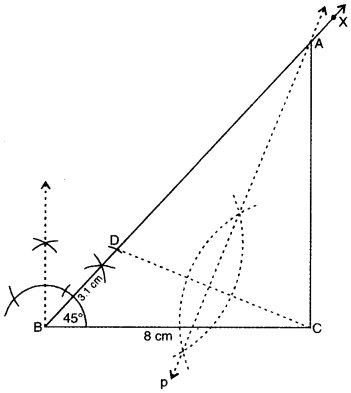Steps of Construction :

1. Draw any line segment BC = 8 cm.
2. At B, construct an angle ∠CBX = 45°.
3. From BX, cut off BD = 3.1 cm.
4. Join DC.
5. Draw the perpendicular bisector ‘p’ of DC and let it intersect BX in A.
6. Join AC. Thus, ∆ABC is the required triangle.

Extra Questions On Constructions Class 9 Question 3.
Construct a ∆ABC such that BC = 3.2 cm, ∠B = 45° and AC – AB = 2.1 cm.
Solution: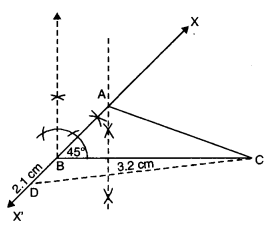Steps of Construction :

1. Draw a line segment BC = 3.2 cm.
2. At B, construct an angle ∠CBX = 45° and produce it to point X’.
3. Cut-off BD = 2.1 cm and join CD.
4. Draw the perpendicular bisector of CD and let it intersect X’BX in A.
5. Join AC. Thus, ∆ABC is the required triangle.

Class 9 Constructions Extra Questions Question 4.
Draw a line segment QR = 5 cm. Construct perpendiculars at point Q and R to it. Name them as QX and RY respectively. Are they both parallel ?
Solution:Steps of Construction :

1. Draw a line segment QR = 5 cm.
2. With Q as centre, construct an angle of 90° and let this line through Q is QX.
3. With R as centre, construct an angle of 90° and let this line through R is RY. Yes, the perpendicular lines QX and RY are parallel.

Class 9 Maths Ch 11 Extra Questions Question 5.
Construct an isosceles triangle whose two equal sides measure 6 cm each and whose base is 5 cm. Draw the perpendicular bisector of its base and show that it passes through the opposite vertex.
Solution:Steps of Construction :

1. Draw a line segment AB = 5 cm.
2. With A and B as centres, draw two arcs of radius 6 cm and let they intersect each other in C.
3. Join AC and BC to get ∆ABC.
4. With A and B as centres, draw two arcs of radius little more than half of AB. Let they intersect each other in P and Q. Join PQ and produce, to pass through C.

### Constructions Class 9 Extra Questions Long Answer Type

Important Questions Of Construction Class 9 Question 1.
Construct a triangle ABC in which BC = 4.7 cm, AB + AC = 8.2 cm and ∠C = 60°.
Solution: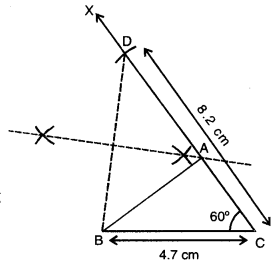Given : In ∆ABC, BC = 4.7 cm, AB + AC = 8.2 cm and ∠C = 60°.
Required : To construct ∆ABC.
Steps of Construction :

1. Draw BC = 4.7 cm.
2. Draw
3. From ray CX, cut off CD = 8.2 cm.
4.  Join BD.
5.  Draw the perpendicular bisector of BD meeting CD at A.
6. Join AB to obtain the required triangle ABC.

Justification :
∵ A lies on the perpendicular bisector of BD, therefore, AB = AD
Now, CD = 8.2 cm
⇒ AC + AD = 8.2 cm
⇒ AC + AB = 8.2 cm.

Class 9th Construction Extra Questions Question 2.
Construct ∆XYZ, if its perimeter is 14 cm, one side of length 5 cm and ∠X = 45°.
Solution: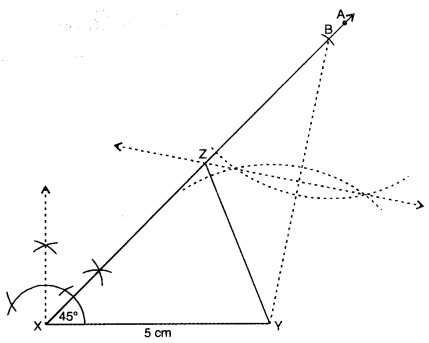Here, perimeter of ∆XYZ = 14 cm and one side XY = 5 cm
∴  YZ + XZ = 14 – 5 = 9 cm and ∠X = 45°.
Steps of Construction :

1. Draw a line segment XY = 5 cm.
2. Construct an ∠YXA = 45° with the help of compass and ruler.
3. From ray XA, cut off XB = 9 cm.
4. Join BY.
5. Draw perpendicular bisector of BY and let it intersect XB in Z.
6. Join ZY. Thus, ∆XYZ is the required triangle.

Extra Questions For Class 9 Maths Constructions Question 3.
To construct a triangle, with perimeter 10 cm and base angles 60° and 45°.
Solution:Given : In ∆ABC,
AB + BC + CA = 10 cm, ∠B = 60° and ∠C = 45°.
Required : To construct ∆ABC.
Steps of Construction :

1. Draw DE = 10 cm.
2. At D, construct ∠EDP= 5 of 60°= 30° and at E, construct DEQ = 1 of 45o = 22°
3. Let DP and EQ meet at A.
4. Draw perpendicular bisector of AD to meet DE at B.
5. Draw perpendicular bisector of AE to meet DE at C.
6. Join AB and AC. Thus, ABC is the required triangle.

### Constructions Class 9 Extra Questions HOTS

Question 1.
Construct an equilateral triangle whose altitude is 6 cm long.
Solution: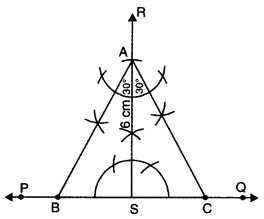Steps of Construction :

1. Draw a line PQ and take any point S on it.
2. Construct the perpendicular SR on PQ.
3. From SR, cut a line segment SA = 6 cm.
4. At the initial point A of the line segment AS, construct ∠SAB = 30° and ∠SAC = 30°.
5. The arms AB and AC of the angles ∠SAB and ∠SAC meet PQ in B and C respectively. Then, ∆ABC is the required equilateral triangle with altitude of length 6 cm.

Question 2.
Construct a rhombus whose diagonals are 8 cm and 6 cm long. Measure the length of each side of the rhombus.
Solution: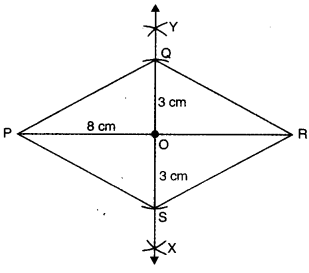Steps of Construction :

1. Draw a line segment PR = 8 cm.
2. Draw the perpendicular bisector XY of the line segment PR. Let O be the point of intersection of PR and XY, so that O is the 8 cm mid-point of PR.
3. From OX, cut a line segment OS = 3 cm and from OY, cut a line segment OQ = 3 cm.
4. Join PS, SR, RQ and QP, then PQRS is the required rhombus.
5. Measure the length of segments PQ, QR, RS and SP, each is found to be 5 cm long.